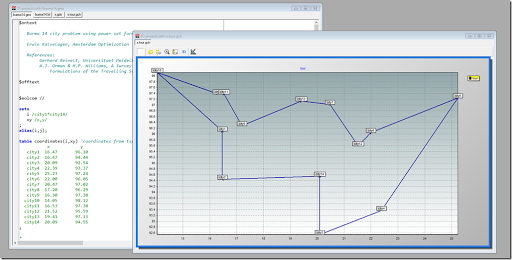## Thursday, February 12, 2009

### TSP Powerset Formulation

> I'm a PhD student in Portugal and I´m modeling a Vehicle Routing
> Problem. I have some difficulties to modelling this subtour
> elimination constraint (n = number of nodes):

> sum(i in S, sum(j in S, x(i,j))) <= |S|-1
> for every nonempty subset S of {2,3,..,n}

This is the Ford, Fulkerson, Johnson (1954) formulation, based on the power of {2,3,..,n}. This generates a lot of constraints (2^(n-1)), with many not active in the optimal solution. So this approach only works for small problems.

 \$ontext   Burma 14 city problem using power set formulation   Erwin Kalvelagen, Amsterdam Optimization   References:        Gerhard Reinelt, Universitaet Heidelberg, TSPLIB95        A.J. Orman & H.P. Williams, A Survey of Different Integer Programming            Formulations of the Travelling Salesman Problem\$offtext\$eolcom //sets   i /city1*city14/   xy /x,y/;alias(i,j);table coordinates(i,xy) 'coordinates from tsplib'           x            y   city1  16.47       96.10   city2  16.47       94.44   city3  20.09       92.54   city4  22.39       93.37   city5  25.23       97.24   city6  22.00       96.05   city7  20.47       97.02   city8  17.20       96.29   city9  16.30       97.38  city10  14.05       98.12  city11  16.53       97.38  city12  21.52       95.59  city13  19.41       97.13  city14  20.09       94.55;** form the distance matrix*parameter latitude(i), longitude(i), degree(i,xy), minute(i,xy);degree(i,xy) = trunc(coordinates(i,xy));minute(i,xy) = coordinates(i,xy) - trunc(coordinates(i,xy));latitude(i) = pi*(degree(i,'x')+5.0*minute(i,'x')/3.0)/180.0;longitude(i) = pi*(degree(i,'y')+5.0*minute(i,'y')/3.0)/180.0;set nd(i,j);nd(i,j)\$(not sameas(i,j)) = yes;scalar rrr /6378.388/;parameter q1(i,j),q2(i,j),q3(i,j);q1(nd(i,j)) = cos(longitude(i) - longitude(j));q2(nd(i,j)) = cos(latitude(i) - latitude(j));q3(nd(i,j)) = cos(latitude(i) + latitude(j));parameter c(i,j) 'distance matrix (KM)';c(nd(i,j)) = trunc(RRR * arccos( 0.5*((1.0+q1(i,j))*q2(i,j) - (1.0-q1(i,j))*q3(i,j)) ) + 1.0);display c;** form the power set*set v(i) 'exclude city 1' /city2*city14/;abort\$(card(i)<>card(v)+1) 'Set v is incorrect',i,v;set n 'subset id (max number of subsets)' /n1*n8192/;scalar nsize 'size needed for set n';nsize = 2**card(v);abort\$(card(n)=2) = yes;** form ps2(n,i,j) = ps(n,i) and ps(n,j)* this will simplify the subtour elimination constraint*alias(v,vv);set ps2(n,i,j);ps2(nsub(n),nd(v,vv))\$(ps(n,v) and ps(n,vv)) = yes;** here is the model*binary variables x(i,j);variable z 'objective variable';equations   obj  'objective'   assign1(i) 'assignment problem constraint'   assign2(j) 'assignment problem constraint'   subt(n)    'subtour elimination';obj..   z =e= sum(nd(i,j), c(i,j)*x(i,j));assign1(i).. sum(nd(i,j), x(i,j)) =e= 1;assign2(j).. sum(nd(i,j), x(i,j)) =e= 1;subt(nsub(n)).. sum(ps2(n,i,j),x(i,j)) =L=  pscount(n)-1;option optcr=0;model tsp /all/;solve tsp minimizing z using mip;

Note: the construct system.powerSetRight is a new GAMS feature (24.0, 2013).

This solves quite fast:

 --- Starting compilation--- burma14.gms(122) 9 Mb--- Starting execution: elapsed 0:00:00.028--- burma14.gms(120) 21 Mb--- Generating MIP model tsp--- burma14.gms(122) 44 Mb---   8,207 rows  183 columns  320,035 non-zeroes---   182 discrete-columns--- Executing CPLEX: elapsed 0:00:00.741 IBM ILOG CPLEX   24.7.3 r58181 Released Jul 11, 2016 WEI x86 64bit/MS Windows --- GAMS/Cplex licensed for continuous and discrete problems.Cplex 12.6.3.0 Reading data...Starting Cplex...Space for names approximately 0.16 MbUse option 'names no' to turn use of names offTried aggregator 1 time.MIP Presolve eliminated 1 rows and 1 columns.Reduced MIP has 8206 rows, 182 columns, and 319852 nonzeros.Reduced MIP has 182 binaries, 0 generals, 0 SOSs, and 0 indicators.Presolve time = 0.28 sec. (95.59 ticks)Probing time = 0.03 sec. (15.13 ticks)Tried aggregator 1 time.Reduced MIP has 8206 rows, 182 columns, and 319852 nonzeros.Reduced MIP has 182 binaries, 0 generals, 0 SOSs, and 0 indicators.Presolve time = 0.28 sec. (95.59 ticks)Probing time = 0.03 sec. (15.13 ticks)Clique table members: 106.MIP emphasis: balance optimality and feasibility.MIP search method: dynamic search.Parallel mode: none, using 1 thread.Tried aggregator 1 time.DUAL formed by presolveReduced LP has 182 rows, 8388 columns, and 320034 nonzeros.Presolve time = 0.08 sec. (27.42 ticks) Iteration log . . .Iteration:     1    Objective     =            70.000000Initializing dual steep norms . . .Root relaxation solution time = 0.16 sec. (57.42 ticks)         Nodes                                         Cuts/   Node  Left     Objective  IInf  Best Integer    Best Bound    ItCnt     Gap *     0     0      integral     0     3323.0000     3323.0000       45    0.00%Elapsed time = 1.30 sec. (460.29 ticks, tree = 0.00 MB, solutions = 1)Found incumbent of value 3323.000000 after 1.30 sec. (460.29 ticks) Root node processing (before b&c):  Real time             =    1.30 sec. (460.33 ticks)Sequential b&c:  Real time             =    0.00 sec. (0.00 ticks)                          ------------Total (root+branch&cut) =    1.30 sec. (460.33 ticks)MIP status(101): integer optimal solutionCplex Time: 1.30sec (det. 460.33 ticks)Fixing integer variables, and solving final LP...Tried aggregator 1 time.LP Presolve eliminated 8207 rows and 183 columns.All rows and columns eliminated.Presolve time = 0.03 sec. (13.14 ticks)Fixed MIP status(1): optimalCplex Time: 0.03sec (det. 17.45 ticks) Proven optimal solution. MIP Solution:         3323.000000    (45 iterations, 0 nodes)Final Solve:          3323.000000    (0 iterations) Best possible:        3323.000000Absolute gap:            0.000000Relative gap:            0.000000 --- Restarting execution--- burma14.gms(122) 20 Mb--- Reading solution for model tsp--- burma14.gms(122) 20 Mb*** Status: Normal completion--- Job burma14.gms Stop 02/23/17 00:47:38 elapsed 0:00:02.719##### References
1. burma14.gms: above model
2. burma14-3.gms: generation of powerset written in GAMS (not using system.PowerSetRight).
3. burma14-2.gms: formulation from Svestka (J.A. Svestka, A continuous variable representation of the TSP, Math Prog, v15, 1978, pp211-213)
4. burma14-4.gms: a cutting plane technique

#### 1 comment:

1.Dear Erwin,

I'm a PhD student working on a Vehicle Routing Problem with Time Windows. I was trying to implement it on GAMS and have difficulties. Then I found the TSP example and would like to ask for help:

(1)
http://www.gams.com/modlib/libhtml/tsp4.htm
Why is the cuts added after the
"solve assign using mip minimizing z;"
line, yet it's still effective in adding cuts into the current model?

(2)
Can you recommend some website / papers where I can finds ways to implement VRP with GAMS? The constraints are really difficult to work with.

Best,

Jim